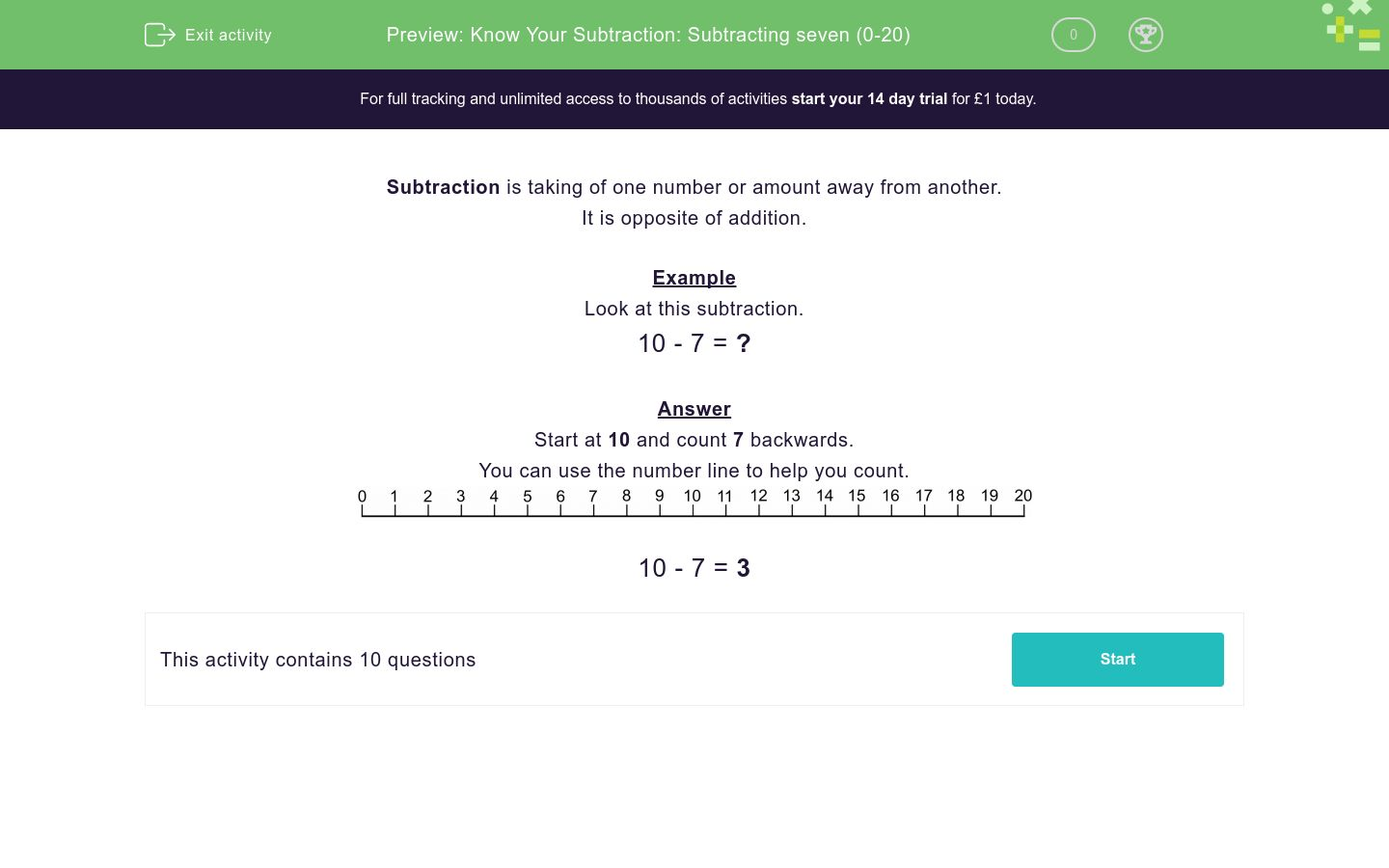# Know Your Subtraction: Subtracting seven (0-20)

In this worksheet, students practise their subtraction using the number 7.Key stage:  KS 1

Curriculum topic:   Number: Addition and Subtraction

Curriculum subtopic:   Add and Subtract to 20

Difficulty level:### QUESTION 1 of 10

Subtraction is taking of one number or amount away from another.

Example

Look at this subtraction.

10 - 7 = ?

Start at 10 and count 7 backwards.10 - 7 = 3

Write the answer to the subtraction.14 - 7 = ?

Write the answer to the subtraction.15 - 7 = ?

Write the answer to the subtraction.13 - 7 = ?

Write the answer to the subtraction.18 - 7 = ?

Write the answer to the subtraction.16 - 7 = ?

Write the answer to the subtraction.12 - 7 = ?

Write the answer to the subtraction.11 - 7 = ?

Write the answer to the subtraction.15 - 7 = ?

Write the answer to the subtraction.20 - 7 = ?

Write the answer to the subtraction.10 - 7 = ?

• Question 1

Write the answer to the subtraction.14 - 7 = ?

7
EDDIE SAYS

Method

14 - 7 = 7

• Question 2

Write the answer to the subtraction.15 - 7 = ?

8
EDDIE SAYS

Method

15 - 7 = 8

• Question 3

Write the answer to the subtraction.13 - 7 = ?

6
EDDIE SAYS

Method

13 - 7 = 6

• Question 4

Write the answer to the subtraction.18 - 7 = ?

11
EDDIE SAYS

Method

18 - 7 = 11

• Question 5

Write the answer to the subtraction.16 - 7 = ?

9
EDDIE SAYS

Method

16 - 7 = 9

• Question 6

Write the answer to the subtraction.12 - 7 = ?

5
EDDIE SAYS

Method

12 - 7 = 5

• Question 7

Write the answer to the subtraction.11 - 7 = ?

4
EDDIE SAYS

Method

11 - 7 = 4

• Question 8

Write the answer to the subtraction.15 - 7 = ?

8
EDDIE SAYS

Method

15 - 7 = 8

• Question 9

Write the answer to the subtraction.20 - 7 = ?

13
EDDIE SAYS

Method

20 - 7 = 13

• Question 10

Write the answer to the subtraction.10 - 7 = ?

3
EDDIE SAYS

Method

10 - 7 = 3

---- OR ----

Sign up for a £1 trial so you can track and measure your child's progress on this activity.

### What is EdPlace?

We're your National Curriculum aligned online education content provider helping each child succeed in English, maths and science from year 1 to GCSE. With an EdPlace account you’ll be able to track and measure progress, helping each child achieve their best. We build confidence and attainment by personalising each child’s learning at a level that suits them.

Get started